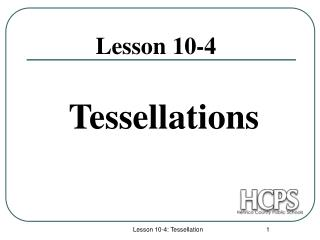DownloadDownload PresentationTessellations

# Tessellations

Download Presentation## Tessellations

- - - - - - - - - - - - - - - - - - - - - - - - - - - E N D - - - - - - - - - - - - - - - - - - - - - - - - - - -
##### Presentation Transcript

1. Tessellations Lesson 10-4 Lesson 10-4: Tessellation

2. Tessellations A tessellation is a design or pattern in which a shape is use repeatedly to cover a plane with no gaps, overlaps, or empty spaces. 1. A regular tessellation is a pattern made with only one type of regular polygon. 2. The sum of the measures surrounding a point (or vertex) must be 360°. 3. Only regular polygons that have an interior angle which is a factor of 360 will tessellate. 4. No regular polygon with more than 6 sides can be used in a regular tessellation. Lesson 10-4: Tessellation

3. Can these figures form a regular tessellation? No. Although this is a regular polygon, it has an interior angle = 135°, which is not a factor of 360 Yes. This is a regular polygon with a 90° interior angle which is a factor of 360. Yes. This is a regular polygon with a 120° interior angle, which is a factor of 360. Lesson 10-4: Tessellation

4. Can these figures form a regular tessellation? Yes. This is a regular polygon with a 60° interior angle which is a factor of 360. No. This is not a regular polygon. It can tessellate but not in a regular tessellation. No. This is not a regular polygon. It can tessellate but not in a regular tessellation. Lesson 10-4: Tessellation

5. How about these for regular tessellation? 1. a 20-sided figure? No, because its interior angle is 162°, which is not a factor of 360. (Interior angle measure : 180(20 - 2) = 162 20 2. a 10-sided figure? No, the interior angle is 144° (not a factor of 360). 3. a 12-sided figure? No, the interior angle is 150° (not a factor of 360). Note: No regular polygon with more than six sides can be used in a regular tessellation. Lesson 10-4: Tessellation

6. Semi-regular Tessellations • If the same combination of regular polygons meet at each vertex, it is called a semi-regular tessellation. Notice the regular octagons with interior angles of 135° and the squares with 90°. At each vertex or point, there is a sum of 135 + 135 + 90 = 360. Lesson 10-4: Tessellation

7. Irregular Tessellations • Other figures can make tessellations which are irregular. The figures used are irregular polygons and may be the same or different types. • Here is an irregular tessellation made with kites and one with trapezoids. Lesson 10-4: Tessellation

8. Make a special tessellation! 1. Begin with a rectangle. 2. Cut a piece out of it and stick on another side. 3. Translate the new figure to create a tessellation. Lesson 10-4: Tessellation

9. Or another . . . • Start with a triangle 2. Cut out a piece of it and slide it to another side. • Slide and reflect the figure repeatedly to create a tessellation. Lesson 10-4: Tessellation

10. Special Notes on Tessellations 1. At each vertex of a tessellation, the sum of the measures of the angles must equal 360. 2. Any quadrilateral will tessellate. 3. Combinations of figures can be used to tessellate. 4. Only equilateral triangles, squares, and regular hexagons can make regular tessellations. Lesson 10-4: Tessellation